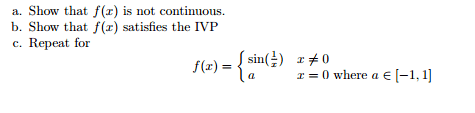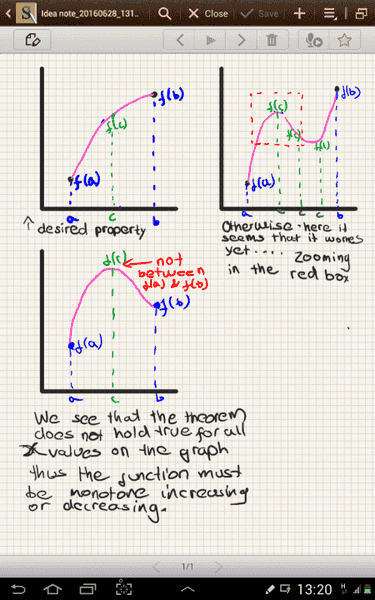# Intermediate Value Property

## Homework Statement'
Here is the given problem

## The Attempt at a Solution

a. For part a, I felt it was not continuous because of the sin(1/x) as it gets closer to 0, the graph switches between 1 and -1. Then I felt it might be continuous, therefore I am not sure.

b. For part b, I felt it has the Intermediate Value Property (IVP), because I can do something with the IVT. Those were my thoughts and ideas.

Last edited by a moderator:

BiGyElLoWhAt
Gold Member
Can you show that the limit as approached positively is different from that as approached negatively? Or that it's different than f(0)? So in other words,
##\lim_{x \to 0} f(x) \neq f(0)##?

For b, I'm not really sure. I thought one of the requirements was that f(x) was continuous...
**
Perhaps this will be of some use.
http://math.stackexchange.com/quest...te-value-property-and-discontinuous-functions
Looks like you need to look at the derivatives near zero.

For part a you would have to do what BiGyElLoWhAt suggested. For b I believe you would have to prove that the function in either monotone increasing or decreasing. IVP says that for any x value between two other x values, the y value will be in between the y values for the other two x values.

Last edited:IVP theorem

Last edited:
pasmith
Homework Helper

## Homework Statement

View attachment 102545'
Here is the given problem

## The Attempt at a Solution

a. For part a, I felt it was not continuous because of the sin(1/x) as it gets closer to 0, the graph switches between 1 and -1. Then I felt it might be continuous, therefore I am not sure.

If there exist sequences $x_n$ and $y_n$ such that $\lim_{n \to \infty} x_n = \lim_{n \to \infty} y_n = 0$ but $\lim_{n \to \infty} f(x_n) \neq \lim_{n \to \infty} f(y_n)$ then $f$ is not continuous at zero.